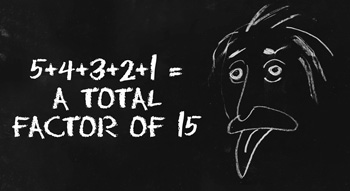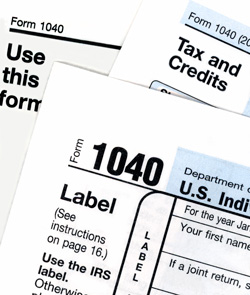# Methods of Asset DepreciationDepreciation is a topic many people find confusing, but the basic concept of depreciation is not particularly complicated. In fact, depreciation is simply a method of allocating the cost of a tangible asset over the expected useful life of the asset. As a simple example, a machine that cost \$1,000 would depreciate at a rate of \$100 per year if its owner projects it will last 10 years. In the real world, depreciation becomes slightly more complicated. Because of the many ways accounting treats depreciation, it can affect both a company’s balance sheet and its income statement. As a result, there are rules governing how to calculate depreciation for particular types of assets and defining the useful life of various assets, and the rules may vary by country or region of the world. In addition, companies may legally use different depreciation calculation methods when calculating income for tax reporting purposes than they do for their financial reports.
• ### Methods of Asset DepreciationThere are many methods of calculating asset depreciation. The simple method used in the example above is called Straight-Line Depreciation. As the name implies, the asset depreciates by the same amount each year until the entire cost has been allocated. Declining Balance Depreciation enables companies to accelerate the depreciation by taking more of the depreciation expense in the early years of the asset’s life. In this method, the company uses a factor and multiplies the straight-line depreciation calculation by that factor. Using the same machine we discussed in the straight-line example above, if the company decided to use the Double Declining Balance method, the first year’s depreciation on the machine would be \$200 not \$100. The catch is that the asset’s value on the balance sheet is now the remaining \$800 and not the \$900 that would have been left using pure straight-line depreciation. For the second year, depreciation would be \$160 and the residual value would be \$740. While the additional depreciation expense helps reduce revenue to lower the company’s tax burden, the decreased asset value reduces the company’s value on the balance sheet. The Units of Production Depreciation method is calculated using the number of times the asset can be operated and attaches a value for each instance. Companies often use this method for tooling or industrial machinery. In this case, assume a particular tool can be used to stamp out 1000 units and the tool itself cost \$1,000. Each time the company uses the tool in the production of product, the company can take \$1 of depreciation expense. The advantage of this method is due to the direct connection between the depreciation expense to the tool’s use in production; it very effectively allocates the expense to the period in which it occurred. The disadvantage is that the method requires careful records of production and usage. Sum of the Years Digits Depreciation is another method of accelerating depreciation. In this case, the years of the asset’s useful life is added to create a factor. For equipment with a 5-year life span, each year’s digit is added (5+4+3+2+1) to create a total factor of 15.To complete the calculation, create a fraction using the actual remaining life expectancy of 5 as the numerator and the sum of 15 as the denominator. In this case, in year one you would be able to depreciate the machine at 5/15 or 1/3. If the machine cost \$600, you would take \$200 of depreciation, using the formula ((600*5)/15)). The asset’s book value would be \$400. For the second year, you would use the fraction 4/15 (remaining years over the sum of the years). That formula would be ((400*4)/15)) and equates to a depreciation expense of slightly more than \$106. These are the most commonly used methods of asset depreciation , and there are variations of each method. Remember the following important points when calculating depreciation:
1. Account for planned salvage value before you calculate depreciation.
2. Be certain the useful life is equal to IRS or local taxing body guidelines when calculating depreciation for tax purposes.
3. Changing depreciation methods during the life of an asset has complex ramifications; it’s best to be sure of your chosen method from the beginning.
• Financial statement impact
Most countries and regions allow companies to calculate different yearly depreciation amounts – one a book depreciation and one a tax depreciation.  A company’s book depreciation expense is the amount recorded on the company’s financial statement and the tax depreciation is recorded on the company’s income tax return.  Keeping these two, different deprecation amounts is an excellent and legal strategy to reduce the amount of taxes the company owes but does require more record keeping.
• Tax ramificationsSince depreciation is an expense, it decreases the company’s taxable income. This may reduce the amount of taxes a company pays. Accelerated depreciation methods allow companies to reduce income in early years, but the tax benefit is offset in later years by the reduced depreciation amounts allowed. Your experienced tax accountant and your business growth strategy may help you decide whether to use accelerated methods. Most taxing authorities, including the IRS, issue guidelines on the useful life of various types of assets, and you should stick to using those guidelines unless you can clearly prove they do not apply.  For example, the IRS requires you to apply the same depreciation method each year of an asset’s lifespan.  Additionally, all assets within the same classification must also be depreciated using the same method. What does this mean?  That you must keep accurate depreciation records for any assets for which you claim tax deductions.  If you are already tracking asset information (location, maintenance, movement, etc.), then it makes sense to use the same asset tracking solution to calculate and record each asset’s yearly depreciation.  Find an asset management solution that allows you to select an appropriate depreciation method for your asset classifications and then calculate – you will then have consistent and auditable reports if they were to ever become necessary.